# 19. Moving Sprites¶

How do we get sprites to move?

To customize our sprite’s behavior, we need to subclass the Sprite class with our own child class. This is easy:

class Coin(arcade.Sprite):


We need to provide each sprite with a update method. The update method is automatically called to update the sprite’s position.

class Coin(arcade.Sprite):

def update(self):
# Code to move goes here


Wait! We have a new class called Coin, but we aren’t using it. Find in our original code this line:

coin = arcade.Sprite("coin_01.png", COIN_SPRITE_SCALING)


See how it is creating an instance of Sprite? We want to create an instance of our new Coin class instead:

coin = Coin("coin_01.png", COIN_SPRITE_SCALING)


Now, how do we get the coin to move?

## 19.1. Moving Sprites Down¶

To get the sprites to “fall” down the screen, we need to make their y location smaller. This is easy. Over-ride update in the sprite and subtract from y each frame:

class Coin(arcade.Sprite):

def update(self):
self.center_y -= 1


Next, create an instance of the Coin class instead of a Sprite class.

Sprite Sample Move Down
  1 2 3 4 5 6 7 8 9 10 11 12 13 14 15 16 17 18 19 20 21 22 23 24 25 26 27 28 29 30 31 32 33 34 35 36 37 38 39 40 41 42 43 44 45 46 47 48 49 50 51 52 53 54 55 56 57 58 59 60 61 62 63 64 65 66 67 68 69 70 71 72 73 74 75 76 77 78 79 80 81 82 83 84 85 86 87 88 89 90 91 92 93 94 95 96 97 98 99 100 101 102 103 104 105 106 107 108 109 110 111 112 113 114 115 """ Sprite Sample Program """ import random import arcade # --- Constants --- SPRITE_SCALING_PLAYER = 0.5 SPRITE_SCALING_COIN = 0.2 COIN_COUNT = 50 SCREEN_WIDTH = 800 SCREEN_HEIGHT = 600 class Coin(arcade.Sprite): def update(self): self.center_y -= 1 class MyGame(arcade.Window): """ Our custom Window Class""" def __init__(self): """ Initializer """ # Call the parent class initializer super().__init__(SCREEN_WIDTH, SCREEN_HEIGHT, "Sprite Example") # Variables that will hold sprite lists self.player_list = None self.coin_list = None # Set up the player info self.player_sprite = None self.score = 0 # Don't show the mouse cursor self.set_mouse_visible(False) arcade.set_background_color(arcade.color.AMAZON) def setup(self): """ Set up the game and initialize the variables. """ # Sprite lists self.player_list = arcade.SpriteList() self.coin_list = arcade.SpriteList() # Score self.score = 0 # Set up the player # Character image from kenney.nl self.player_sprite = arcade.Sprite("character.png", SPRITE_SCALING_PLAYER) self.player_sprite.center_x = 50 self.player_sprite.center_y = 50 self.player_list.append(self.player_sprite) # Create the coins for i in range(COIN_COUNT): # Create the coin instance # Coin image from kenney.nl coin = Coin("coin_01.png", SPRITE_SCALING_COIN) # Position the coin coin.center_x = random.randrange(SCREEN_WIDTH) coin.center_y = random.randrange(SCREEN_HEIGHT) # Add the coin to the lists self.coin_list.append(coin) def on_draw(self): """ Draw everything """ arcade.start_render() self.coin_list.draw() self.player_list.draw() # Put the text on the screen. output = f"Score: {self.score}" arcade.draw_text(output, 10, 20, arcade.color.WHITE, 14) def on_mouse_motion(self, x, y, dx, dy): """ Handle Mouse Motion """ # Move the center of the player sprite to match the mouse x, y self.player_sprite.center_x = x self.player_sprite.center_y = y def update(self, delta_time): """ Movement and game logic """ # Call update on all sprites (The sprites don't do much in this # example though.) self.coin_list.update() # Generate a list of all sprites that collided with the player. hit_list = arcade.check_for_collision_with_list(self.player_sprite, self.coin_list) # Loop through each colliding sprite, remove it, and add to the score. for coin in hit_list: coin.remove_from_sprite_lists() self.score += 1 def main(): """ Main method """ window = MyGame() window.setup() arcade.run() if __name__ == "__main__": main() 

This causes the coins to move down. But once they move off the screen they keep going into negative-coordinate land. We can’t see them any more. Sad.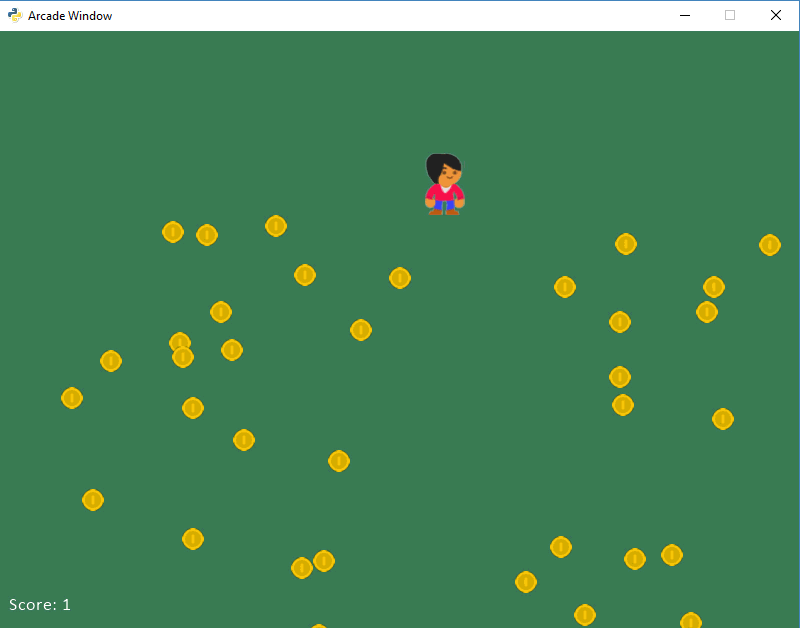Coins moving down

### 19.1.1. Resetting to the Top¶

We can get around this by resetting the coins up to the top. Here’s how its done:

class Coin(arcade.Sprite):

def update(self):
self.center_y -= 1

# See if we went off-screen
if self.center_y < 0:
self.center_y = SCREEN_HEIGHT


But this isn’t perfect. Because if your eyes are fast, you can see the coin ‘pop’ in and out of existence at the edge. It doesn’t smoothly slide off. This is because we move it when the center of the coin is at the edge. Not the top of the coin has slid off.

There are a couple ways we can do this. Here’s one. We’ll check at -20 instead of 0. As long as the coin radius is 20 or less, we are good.

class Coin(arcade.Sprite):

def update(self):
self.center_y -= 1

# See if we went off-screen
if self.center_y < -20:
self.center_y = SCREEN_HEIGHT + 20


There’s another way. In addition to center_y, sprites have other members that are useful in these cases. They are top, bottom, left and right. So we can do this:

class Coin(arcade.Sprite):

def update(self):
self.center_y -= 1

# See if we went off-screen
if self.top < 0:
self.bottom = SCREEN_HEIGHT


Doing this allows the coins to smoothly slide on and off the screen. But since they reappear at the top, we get repeating patters. See the image below: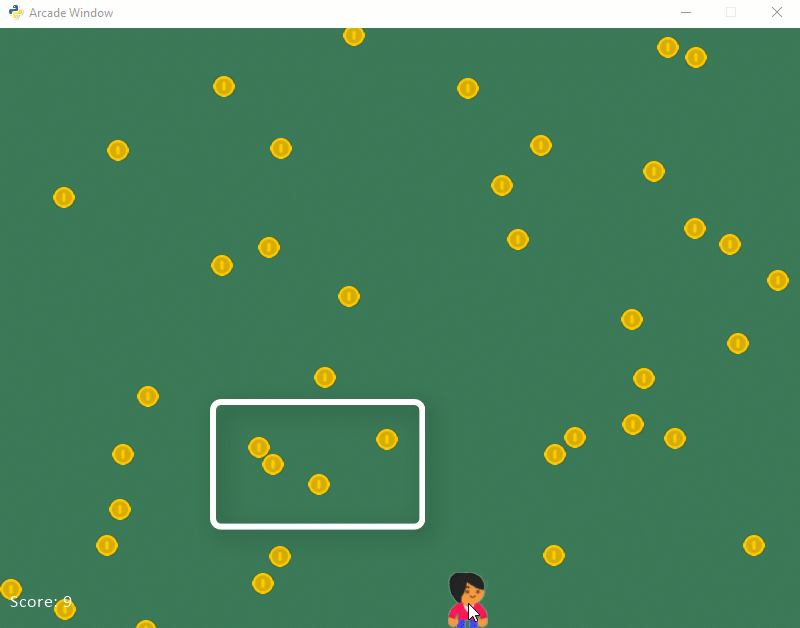Coins repeating in a pattern

Instead we can randomize it a bit:

def update(self):

# Move the coin
self.center_y -= 1

# See if the coin has fallen off the bottom of the screen.
# If so, reset it.
if self.top < 0:
# Reset the coin to a random spot above the screen
self.center_y = random.randrange(SCREEN_HEIGHT + 20,
SCREEN_HEIGHT + 100)
self.center_x = random.randrange(SCREEN_WIDTH)


### 19.1.2. Never Ending Coins¶

This works, but when we we collect all the coins we are done. What if it was a never-ending set of coins? Instead of “killing” the coin, let’s reset it to the top of the screen.

def update(self, delta_time):
""" Movement and game logic """

self.coin_list.update()

for coin in hit_list:
self.score += 1

# Reset the coin to a random spot above the screen
coin.center_y = random.randrange(SCREEN_HEIGHT + 20,
SCREEN_HEIGHT + 100)
coin.center_x = random.randrange(SCREEN_WIDTH)


We can even take that common code, and move it to a method. Here’s a full example:

Full Move Down Sprite Sample
  1 2 3 4 5 6 7 8 9 10 11 12 13 14 15 16 17 18 19 20 21 22 23 24 25 26 27 28 29 30 31 32 33 34 35 36 37 38 39 40 41 42 43 44 45 46 47 48 49 50 51 52 53 54 55 56 57 58 59 60 61 62 63 64 65 66 67 68 69 70 71 72 73 74 75 76 77 78 79 80 81 82 83 84 85 86 87 88 89 90 91 92 93 94 95 96 97 98 99 100 101 102 103 104 105 106 107 108 109 110 111 112 113 114 115 116 117 118 119 120 121 122 123 124 125 126 127 128 129 130 131 132 133 134 """ Sprite Sample Program """ import random import arcade # --- Constants --- SPRITE_SCALING_PLAYER = 0.5 SPRITE_SCALING_COIN = 0.2 COIN_COUNT = 50 SCREEN_WIDTH = 800 SCREEN_HEIGHT = 600 class Coin(arcade.Sprite): """ This class represents the coins on our screen. It is a child class of the arcade library's "Sprite" class. """ def reset_pos(self): # Reset the coin to a random spot above the screen self.center_y = random.randrange(SCREEN_HEIGHT + 20, SCREEN_HEIGHT + 100) self.center_x = random.randrange(SCREEN_WIDTH) def update(self): # Move the coin self.center_y -= 1 # See if the coin has fallen off the bottom of the screen. # If so, reset it. if self.top < 0: self.reset_pos() class MyGame(arcade.Window): """ Our custom Window Class""" def __init__(self): """ Initializer """ # Call the parent class initializer super().__init__(SCREEN_WIDTH, SCREEN_HEIGHT, "Sprite Example") # Variables that will hold sprite lists self.player_list = None self.coin_list = None # Set up the player info self.player_sprite = None self.score = 0 # Don't show the mouse cursor self.set_mouse_visible(False) arcade.set_background_color(arcade.color.AMAZON) def setup(self): """ Set up the game and initialize the variables. """ # Sprite lists self.player_list = arcade.SpriteList() self.coin_list = arcade.SpriteList() # Score self.score = 0 # Set up the player # Character image from kenney.nl self.player_sprite = arcade.Sprite("character.png", SPRITE_SCALING_PLAYER) self.player_sprite.center_x = 50 self.player_sprite.center_y = 50 self.player_list.append(self.player_sprite) # Create the coins for i in range(COIN_COUNT): # Create the coin instance # Coin image from kenney.nl coin = Coin("coin_01.png", SPRITE_SCALING_COIN) # Position the coin coin.center_x = random.randrange(SCREEN_WIDTH) coin.center_y = random.randrange(SCREEN_HEIGHT) # Add the coin to the lists self.coin_list.append(coin) def on_draw(self): """ Draw everything """ arcade.start_render() self.coin_list.draw() self.player_list.draw() # Put the text on the screen. output = f"Score: {self.score}" arcade.draw_text(output, 10, 20, arcade.color.WHITE, 14) def on_mouse_motion(self, x, y, dx, dy): """ Handle Mouse Motion """ # Move the center of the player sprite to match the mouse x, y self.player_sprite.center_x = x self.player_sprite.center_y = y def update(self, delta_time): """ Movement and game logic """ # Call update on all sprites (The sprites don't do much in this # example though.) self.coin_list.update() # Generate a list of all sprites that collided with the player. hit_list = arcade.check_for_collision_with_list(self.player_sprite, self.coin_list) # Loop through each colliding sprite, remove it, and add to the score. for coin in hit_list: coin.remove_from_sprite_lists() self.score += 1 def main(): """ Main method """ window = MyGame() window.setup() arcade.run() if __name__ == "__main__": main() 

## 19.2. Bouncing Coins¶

Instead of always adding one to the y-coordinate and have the sprites move down, we can keep a vector by using change_x and change_y. By using these, we can have the sprite bounce around the screen: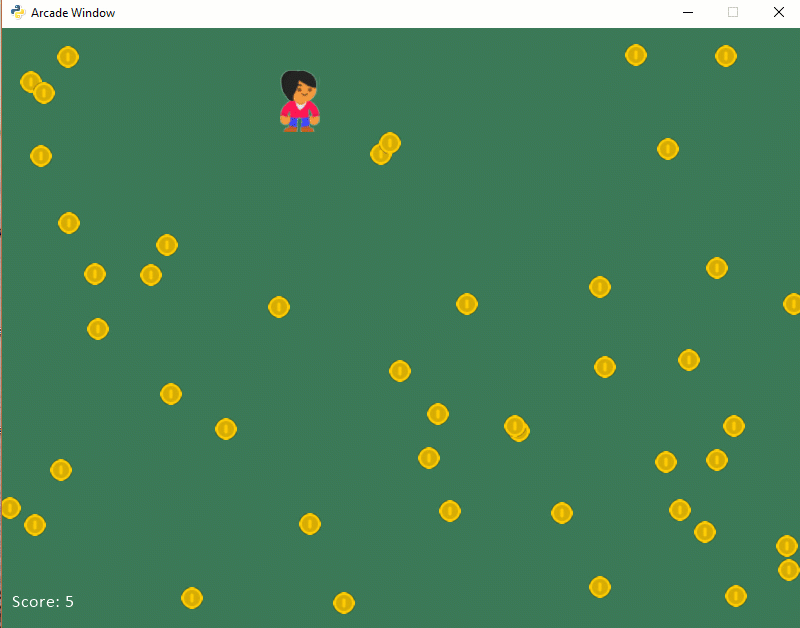Coins bouncing around

sprites_sample_move_bouncing.py
  1 2 3 4 5 6 7 8 9 10 11 12 13 14 15 16 17 18 19 20 21 22 23 24 25 26 27 28 29 30 31 32 33 34 35 36 37 38 39 40 41 42 43 44 45 46 47 48 49 50 51 52 53 54 55 56 57 58 59 60 61 62 63 64 65 66 67 68 69 70 71 72 73 74 75 76 77 78 79 80 81 82 83 84 85 86 87 88 89 90 91 92 93 94 95 96 97 98 99 100 101 102 103 104 105 106 107 108 109 110 111 112 113 114 115 116 117 118 119 120 121 122 123 124 125 126 127 128 129 130 131 132 133 134 135 136 137 138 139 140 """ Sprite Sample Program """ import random import arcade # --- Constants --- SPRITE_SCALING_PLAYER = 0.5 SPRITE_SCALING_COIN = 0.2 COIN_COUNT = 50 SCREEN_WIDTH = 800 SCREEN_HEIGHT = 600 class Coin(arcade.Sprite): def __init__(self, filename, sprite_scaling): super().__init__(filename, sprite_scaling) self.change_x = 0 self.change_y = 0 def update(self): # Move the coin self.center_x += self.change_x self.center_y += self.change_y # If we are out-of-bounds, then 'bounce' if self.left < 0: self.change_x *= -1 if self.right > SCREEN_WIDTH: self.change_x *= -1 if self.bottom < 0: self.change_y *= -1 if self.top > SCREEN_HEIGHT: self.change_y *= -1 class MyGame(arcade.Window): """ Our custom Window Class""" def __init__(self): """ Initializer """ # Call the parent class initializer super().__init__(SCREEN_WIDTH, SCREEN_HEIGHT, "Sprite Example") # Variables that will hold sprite lists self.player_list = None self.coin_list = None # Set up the player info self.player_sprite = None self.score = 0 # Don't show the mouse cursor self.set_mouse_visible(False) arcade.set_background_color(arcade.color.AMAZON) def setup(self): """ Set up the game and initialize the variables. """ # Sprite lists self.player_list = arcade.SpriteList() self.coin_list = arcade.SpriteList() # Score self.score = 0 # Set up the player # Character image from kenney.nl self.player_sprite = arcade.Sprite("character.png", SPRITE_SCALING_PLAYER) self.player_sprite.center_x = 50 self.player_sprite.center_y = 50 self.player_list.append(self.player_sprite) # Create the coins for i in range(COIN_COUNT): # Create the coin instance # Coin image from kenney.nl coin = Coin("coin_01.png", SPRITE_SCALING_COIN) # Position the coin coin.center_x = random.randrange(SCREEN_WIDTH) coin.center_y = random.randrange(SCREEN_HEIGHT) coin.change_x = random.randrange(-3, 4) coin.change_y = random.randrange(-3, 4) # Add the coin to the lists self.coin_list.append(coin) def on_draw(self): """ Draw everything """ arcade.start_render() self.coin_list.draw() self.player_list.draw() # Put the text on the screen. output = f"Score: {self.score}" arcade.draw_text(output, 10, 20, arcade.color.WHITE, 14) def on_mouse_motion(self, x, y, dx, dy): """ Handle Mouse Motion """ # Move the center of the player sprite to match the mouse x, y self.player_sprite.center_x = x self.player_sprite.center_y = y def update(self, delta_time): """ Movement and game logic """ # Call update on all sprites (The sprites don't do much in this # example though.) self.coin_list.update() # Generate a list of all sprites that collided with the player. hit_list = arcade.check_for_collision_with_list(self.player_sprite, self.coin_list) # Loop through each colliding sprite, remove it, and add to the score. for coin in hit_list: coin.remove_from_sprite_lists() self.score += 1 def main(): """ Main method """ window = MyGame() window.setup() arcade.run() if __name__ == "__main__": main() 

TODO: Put in some text about spawning a sprite too close to the edge. Also make a refer to it from the final project.

Take what you’ve learned from the example above, and see if you can replicate this: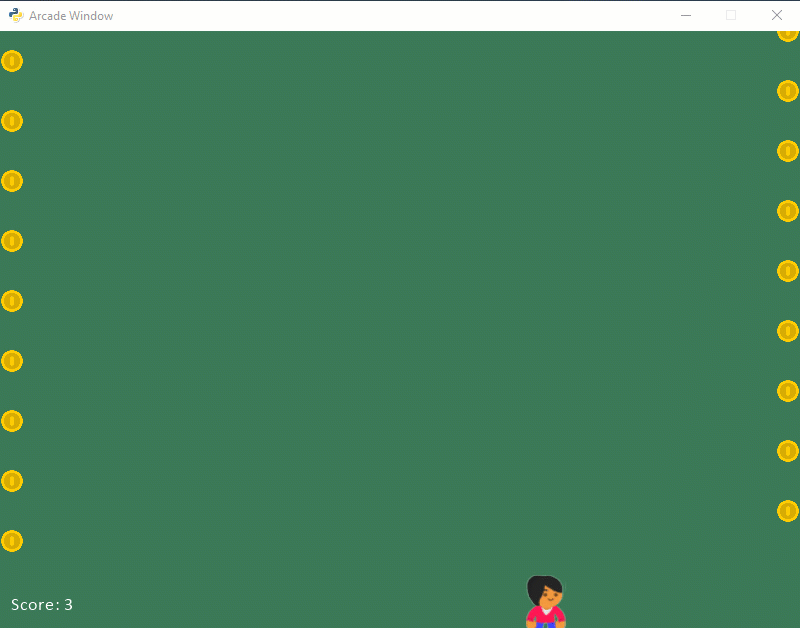Test Pattern

## 19.3. Coins Moving In Circles¶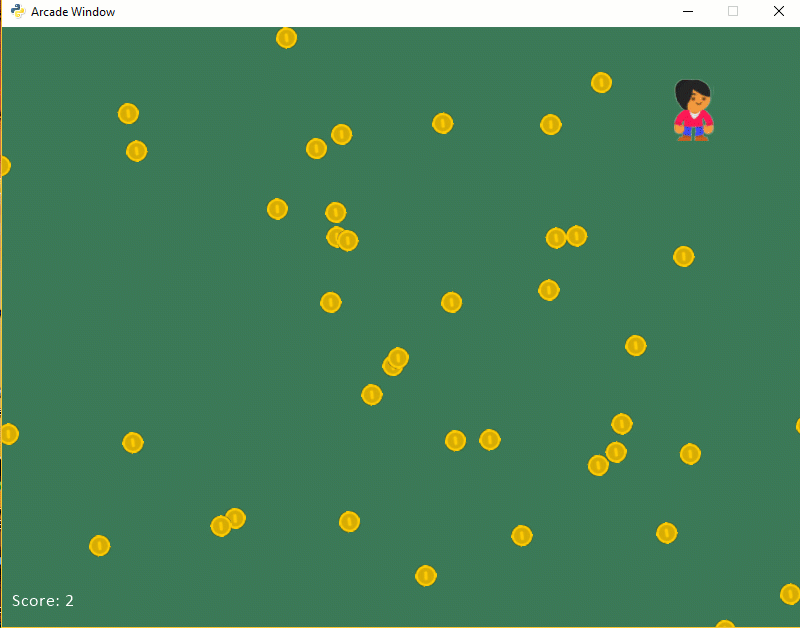Coins moving in a circle

sprites_circle.py
  1 2 3 4 5 6 7 8 9 10 11 12 13 14 15 16 17 18 19 20 21 22 23 24 25 26 27 28 29 30 31 32 33 34 35 36 37 38 39 40 41 42 43 44 45 46 47 48 49 50 51 52 53 54 55 56 57 58 59 60 61 62 63 64 65 66 67 68 69 70 71 72 73 74 75 76 77 78 79 80 81 82 83 84 85 86 87 88 89 90 91 92 93 94 95 96 97 98 99 100 101 102 103 104 105 106 107 108 109 110 111 112 113 114 115 116 117 118 119 120 121 122 123 124 125 126 127 128 129 130 131 132 133 134 135 136 137 138 139 140 141 import random import arcade import math SPRITE_SCALING = 0.5 SCREEN_WIDTH = 800 SCREEN_HEIGHT = 600 class Coin(arcade.Sprite): def __init__(self, filename, sprite_scaling): """ Constructor. """ # Call the parent class (Sprite) constructor super().__init__(filename, sprite_scaling) # Current angle in radians self.circle_angle = 0 # How far away from the center to orbit, in pixels self.circle_radius = 0 # How fast to orbit, in radians per frame self.circle_speed = 0.008 # Set the center of the point we will orbit around self.circle_center_x = 0 self.circle_center_y = 0 def update(self): """ Update the ball's position. """ # Calculate a new x, y self.center_x = self.circle_radius * math.sin(self.circle_angle) \ + self.circle_center_x self.center_y = self.circle_radius * math.cos(self.circle_angle) \ + self.circle_center_y # Increase the angle in prep for the next round. self.circle_angle += self.circle_speed class MyGame(arcade.Window): """ Main application class. """ def __init__(self, width, height): super().__init__(width, height) # Sprite lists self.player_list = None self.coin_list = None # Set up the player self.score = 0 self.player_sprite = None def start_new_game(self): """ Set up the game and initialize the variables. """ # Sprite lists self.player_list = arcade.SpriteList() self.coin_list = arcade.SpriteList() # Set up the player self.score = 0 # Character image from kenney.nl self.player_sprite = arcade.Sprite("character.png", SPRITE_SCALING) self.player_sprite.center_x = 50 self.player_sprite.center_y = 70 self.player_list.append(self.player_sprite) for i in range(50): # Create the coin instance # Coin image from kenney.nl coin = Coin("coin_01.png", SPRITE_SCALING / 3) # Position the center of the circle the coin will orbit coin.circle_center_x = random.randrange(SCREEN_WIDTH) coin.circle_center_y = random.randrange(SCREEN_HEIGHT) # Random radius from 10 to 200 coin.circle_radius = random.randrange(10, 200) # Random start angle from 0 to 2pi coin.circle_angle = random.random() * 2 * math.pi # Add the coin to the lists self.coin_list.append(coin) # Don't show the mouse cursor self.set_mouse_visible(False) # Set the background color arcade.set_background_color(arcade.color.AMAZON) def on_draw(self): # This command has to happen before we start drawing arcade.start_render() # Draw all the sprites. self.coin_list.draw() self.player_list.draw() # Put the text on the screen. output = "Score: " + str(self.score) arcade.draw_text(output, 10, 20, arcade.color.WHITE, 14) def on_mouse_motion(self, x, y, dx, dy): self.player_sprite.center_x = x self.player_sprite.center_y = y def update(self, delta_time): """ Movement and game logic """ # Call update on all sprites (The sprites don't do much in this # example though.) self.coin_list.update() # Generate a list of all sprites that collided with the player. hit_list = arcade.check_for_collision_with_list(self.player_sprite, self.coin_list) # Loop through each colliding sprite, remove it, and add to the score. for coin in hit_list: self.score += 1 coin.remove_from_sprite_lists() def main(): window = MyGame(SCREEN_WIDTH, SCREEN_HEIGHT) window.start_new_game() arcade.run() if __name__ == "__main__": main() 

Sprites can easily be rotated by setting their angle attribute. Angles are in degrees. So the following will flip the player upside down:

self.player_sprite.angle = 180


If you put this in the coin’s update method, it would cause the coins to spin:

self.angle += 1

# If we rotate past 360, reset it back a turn.
if self.angle > 359:
self.angle -= 360